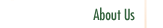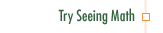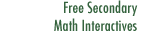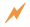Linear Equations

Most algebra curricula introduce linear equations before linear functions. In Linear Equations, functions are discussed first. Learners conceive and manipulate equations as particular values of a general pattern—functions

Professional Development Goals

• Understand the rationale behind the rules of symbol manipulation that maintain an equality or corresponding inequality
• Deepen the distinction between equivalence of function and equality of value
• Gain facility in moving easily between symbolic and graphic techniques for solving equations and inequalities, whether presented in symbolic or story (text) formClick to Get Seeing Math™ SecondaryCourse Listing Proportional Reasoning Linear Functions Transformations of Linear Functions Linear Equations Systems of Linear Equations Quadratic Functions Transformations of Quadratic Functions Quadratic Equations Data Analysis